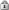11Dimensions | Why 11D ?The company name “11 Dimensions” is derived from a mind game.

We would like to invite you to this mind game as follows:

Two Dimensions

Imagine a three-dimensional six-sided cube with three equal sides (Laplace cube). If one were to draw this cube on a sheet of paper, one would project it into the second dimension – as a two-dimensional diagram. It is clear to us all that we lose something of the third dimension in doing so, provided that we do not draw more than one diagram.

Four Dimensions

Let us take this three-dimensional cube and throw it through space from point A to point B. Some time will elapse, before the cube reaches point B, i.e. one could say we move the cube in “time” or, in other words, in the fourth dimension. We can all envisage the route described by a cube traveling from A to B. This route could be seen as representing the fourth dimension. If we now observe the cube at a given point in time t each time it is in a dimensional cube c(t).

Then we take the cube c in point A and the cube c in point B and connect the corners. We could also envisage this dimension as a tube x. Now we should depart from our normal method of perception.

More Dimensions

We now go into the fifth dimension and take tube x, copy it and move it to x` and connect the corners. This gives us a kind of airbed. a. But what is this airbed a? First we must admit that we were observing everything from aspect of the third dimension, even with the tube example of the fourth dimension. We thought of the fifth dimension as a way of describing a further possibility. In the same way we were able to imagine the other known dimensions as possibilities. We normally would not do so, since they appear to us as part of “reality”, but we could do this, since an object (3d) is a possibility in time. Similarly a line or plane is too, so we see that the smaller the dimension becomes in which we find ourselves, the more possibilities there are.Legal | Privacy statement | Using this site means you accept its terms | Sitemap | Home